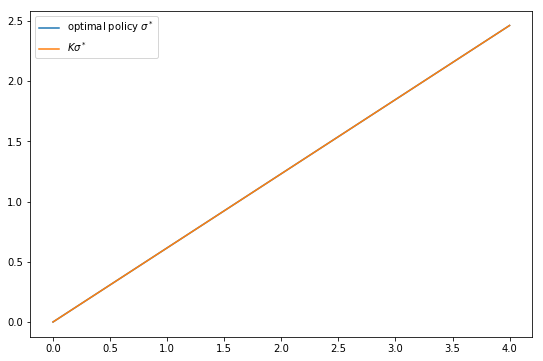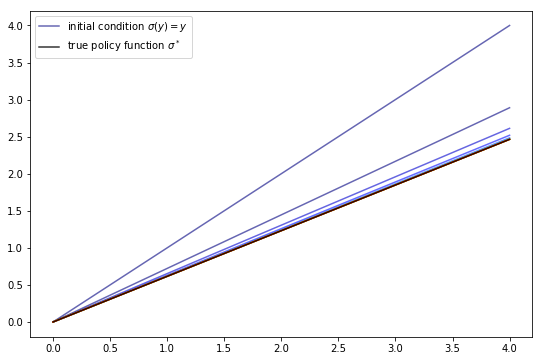## Download PDF

How to read this lecture...

Code should execute sequentially if run in a Jupyter notebook

# Optimal Growth III: The Endogenous Grid Method¶

## Contents¶

In addition to what’s in Anaconda, this lecture will need the following libraries:

In :
!pip install --upgrade quantecon
!pip install interpolation


## Overview¶

We solved the stochastic optimal growth model using

We found time iteration to be significantly more accurate at each step.

In this lecture, we’ll look at an ingenious twist on the time iteration technique called the endogenous grid method (EGM).

EGM is a numerical method for implementing policy iteration invented by Chris Carroll.

It is a good example of how a clever algorithm can save a massive amount of computer time.

(Massive when we multiply saved CPU cycles on each implementation times the number of implementations worldwide)

The original reference is [Car06].

Let’s start with some imports

In :
import numpy as np
import quantecon as qe
from interpolation import interp
from numba import njit, prange
from quantecon.optimize import brentq
import matplotlib.pyplot as plt
%matplotlib inline


## Key Idea¶

Let’s start by reminding ourselves of the theory and then see how the numerics fit in.

### Theory¶

Take the model set out in the time iteration lecture, following the same terminology and notation.

The Euler equation is

$$(u'\circ \sigma^*)(y) = \beta \int (u'\circ \sigma^*)(f(y - \sigma^*(y)) z) f'(y - \sigma^*(y)) z \phi(dz) \tag{1}$$

As we saw, the Coleman-Reffett operator is a nonlinear operator $K$ engineered so that $\sigma^*$ is a fixed point of $K$.

It takes as its argument a continuous strictly increasing consumption policy $\sigma \in \Sigma$.

It returns a new function $K \sigma$, where $(K \sigma)(y)$ is the $c \in (0, \infty)$ that solves

$$u'(c) = \beta \int (u' \circ \sigma) (f(y - c) z ) f'(y - c) z \phi(dz) \tag{2}$$

### Exogenous Grid¶

As discussed in the lecture on time iteration, to implement the method on a computer we need a numerical approximation.

In particular, we represent a policy function by a set of values on a finite grid.

The function itself is reconstructed from this representation when necessary, using interpolation or some other method.

Previously, to obtain a finite representation of an updated consumption policy we

• fixed a grid of income points $\{y_i\}$
• calculated the consumption value $c_i$ corresponding to each $y_i$ using (2) and a root-finding routine

Each $c_i$ is then interpreted as the value of the function $K \sigma$ at $y_i$.

Thus, with the points $\{y_i, c_i\}$ in hand, we can reconstruct $K \sigma$ via approximation.

Iteration then continues…

### Endogenous Grid¶

The method discussed above requires a root-finding routine to find the $c_i$ corresponding to a given income value $y_i$.

Root-finding is costly because it typically involves a significant number of function evaluations.

As pointed out by Carroll [Car06], we can avoid this if $y_i$ is chosen endogenously.

The only assumption required is that $u'$ is invertible on $(0, \infty)$.

The idea is this:

First, we fix an exogenous grid $\{k_i\}$ for capital ($k = y - c$).

Then we obtain $c_i$ via

$$c_i = (u')^{-1} \left\{ \beta \int (u' \circ \sigma) (f(k_i) z ) \, f'(k_i) \, z \, \phi(dz) \right\} \tag{3}$$

where $(u')^{-1}$ is the inverse function of $u'$.

Finally, for each $c_i$ we set $y_i = c_i + k_i$.

It is clear that each $(y_i, c_i)$ pair constructed in this manner satisfies (2).

With the points $\{y_i, c_i\}$ in hand, we can reconstruct $K \sigma$ via approximation as before.

The name EGM comes from the fact that the grid $\{y_i\}$ is determined endogenously.

## Implementation¶

Let’s implement this version of the Coleman-Reffett operator and see how it performs.

First, we will construct a class OptimalGrowthModel to hold the parameters of the model.

In :
class OptimalGrowthModel:

"""

The class holds parameters and true value and policy functions.
"""

def __init__(self,
f,                # Production function
f_prime,          # f'(k)
u,                # Utility function
u_prime,          # Marginal utility
u_prime_inv,      # Inverse marginal utility
β=0.96,           # Discount factor
μ=0,
s=0.1,
grid_max=4,
grid_size=200,
shock_size=250):

self.β, self.μ, self.s = β, μ, s
self.f, self.u = f, u
self.f_prime, self.u_prime, self.u_prime_inv = f_prime, u_prime, u_prime_inv

self.grid = np.linspace(1e-5, grid_max, grid_size)         # Set up grid
self.shocks = np.exp(μ + s * np.random.randn(shock_size))  # Store shocks


### The Operator¶

Here’s an implementation of $K$ using EGM as described above.

Unlike the previous lecture, we do not just-in-time compile the operator because we want to return the policy function.

Despite this, the EGM method is still faster than the standard Coleman-Reffett operator, as we will see later on.

In :
def egm_operator_factory(og):
"""
A function factory for building the Coleman-Reffett operator

Here og is an instance of OptimalGrowthModel.
"""

f, u, β = og.f, og.u, og.β
f_prime, u_prime, u_prime_inv = og.f_prime, og.u_prime, og.u_prime_inv
grid, shocks = og.grid, og.shocks

def K(σ):
"""
The Bellman operator

* σ is a function
"""
# Allocate memory for value of consumption on endogenous grid points
c = np.empty_like(grid)

# Solve for updated consumption value
for i, k in enumerate(grid):
vals = u_prime(σ(f(k) * shocks)) * f_prime(k) * shocks
c[i] = u_prime_inv(β * np.mean(vals))

# Determine endogenous grid
y = grid + c  # y_i = k_i + c_i

# Update policy function and return
σ_new = lambda x: interp(y, c, x)

return σ_new

return K


Note the lack of any root-finding algorithm.

We’ll also run our original implementation, which uses an exogenous grid and requires root-finding, so we can perform some comparisons.

In :
def time_operator_factory(og, parallel_flag=True):
"""
A function factory for building the Coleman-Reffett operator.
Here og is an instance of OptimalGrowthModel.
"""
β = og.β
f, u = og.f, og.u
f_prime, u_prime = og.f_prime, og.u_prime
grid, shocks = og.grid, og.shocks

@njit
def objective(c, σ, y):
"""
The right hand side of the operator
"""
# First turn w into a function via interpolation
σ_func = lambda x: interp(grid, σ, x)
vals = u_prime(σ_func(f(y - c) * shocks)) * f_prime(y - c) * shocks
return u_prime(c) - β * np.mean(vals)

@njit(parallel=parallel_flag)
def K(σ):
"""
The Coleman-Reffett operator
"""
σ_new = np.empty_like(σ)
for i in prange(len(grid)):
y = grid[i]
# Solve for optimal c at y
c_star = brentq(objective, 1e-10, y-1e-10, args=(σ, y))
σ_new[i] = c_star

return σ_new

return K


Let’s test out the code above on some example parameterizations.

### Testing on the Log / Cobb–Douglas Case¶

As we did for value function iteration and time iteration, let’s start by testing our method with the log-linear benchmark.

First, we generate an instance

In :
α = 0.4  # Production function parameter

@njit
def f(k):
"""
Cobb-Douglas production function
"""
return k**α

@njit
def f_prime(k):
"""
First derivative of the production function
"""
return α * k**(α - 1)

@njit
def u_prime(c):
return 1 / c

og = OptimalGrowthModel(f=f,
f_prime=f_prime,
u=np.log,
u_prime=u_prime,
u_prime_inv=u_prime)


Notice that we’re passing u_prime twice.

The reason is that, in the case of log utility, $u'(c) = (u')^{-1}(c) = 1/c$.

Hence u_prime and u_prime_inv are the same.

As a preliminary test, let’s see if $K \sigma^* = \sigma^*$, as implied by the theory

In :
β, grid = og.β, og.grid

def c_star(y):
"True optimal policy"
return (1 - α * β) * y

K = egm_operator_factory(og)  # Return the operator K with endogenous grid

fig, ax = plt.subplots(figsize=(9, 6))

ax.plot(grid, c_star(grid), label="optimal policy $\sigma^*$")
ax.plot(grid, K(c_star)(grid), label="$K\sigma^*$")

ax.legend()
plt.show()We can’t really distinguish the two plots.

In fact it’s easy to see that the difference is essentially zero:

In :
max(abs(K(c_star)(grid) - c_star(grid)))

Out:
9.881666666666669e-06

Next, let’s try iterating from an arbitrary initial condition and see if we converge towards $\sigma^*$.

Let’s start from the consumption policy that eats the whole pie: $\sigma(y) = y$

In :
σ = lambda x: x
n = 15
fig, ax = plt.subplots(figsize=(9, 6))

ax.plot(grid, σ(grid), color=plt.cm.jet(0),
alpha=0.6, label='initial condition $\sigma(y) = y$')

for i in range(n):
σ = K(σ)  # Update policy
ax.plot(grid, σ(grid), color=plt.cm.jet(i / n),  alpha=0.6)

ax.plot(grid, c_star(grid), 'k-',
alpha=0.8, label='true policy function $\sigma^*$')

ax.legend()
plt.show()We see that the policy has converged nicely, in only a few steps.

## Speed¶

Now let’s compare the clock times per iteration for the standard Coleman-Reffett operator (with exogenous grid) and the EGM version.

We’ll do so using the CRRA model adopted in the exercises of the Euler equation time iteration lecture.

In :
γ = 1.5   # Preference parameter

@njit
def u(c):
return (c**(1 - γ) - 1) / (1 - γ)

@njit
def u_prime(c):
return c**(-γ)

@njit
def u_prime_inv(c):
return c**(-1 / γ)

og = OptimalGrowthModel(f=f,
f_prime=f_prime,
u=u,
u_prime=u_prime,
u_prime_inv=u_prime_inv)

K_time = time_operator_factory(og)     # Standard Coleman-Reffett operator
K_time(grid)                           # Call once to compile jitted version
K_egm = egm_operator_factory(og)       # Coleman-Reffett operator with endogenous grid


Here’s the result

In :
sim_length = 20

print("Timing standard Coleman policy function iteration")
σ = grid    # Initial policy
qe.util.tic()
for i in range(sim_length):
σ_new = K_time(σ)
σ = σ_new
qe.util.toc()

print("Timing policy function iteration with endogenous grid")
σ = lambda x: x  # Initial policy
qe.util.tic()
for i in range(sim_length):
σ_new = K_egm(σ)
σ = σ_new
qe.util.toc()

Timing standard Coleman policy function iteration
TOC: Elapsed: 0:00:0.28
Timing policy function iteration with endogenous grid
TOC: Elapsed: 0:00:0.23

Out:
0.23688840866088867

We see that the EGM version is significantly faster, even without jit compilation!

The absence of numerical root-finding means that it is typically more accurate at each step as well.

• Share page